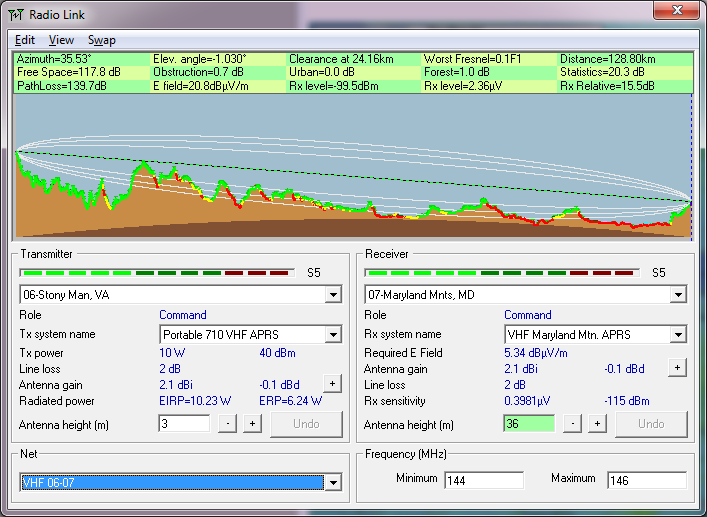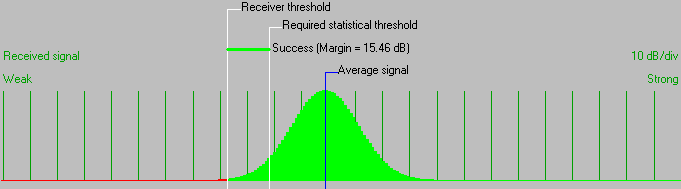• Distance between 06-Stony Man, VA and 07-Maryland Mnts, MD is 128.8 km (80.0 miles)
• True North Azimuth = 35.53°, Magnetic North Azimuth = 45.33°, Elevation angle = -1.0297°
• Terrain elevation variation is 1134.5 m
• Propagation mode is line-of-sight, minimum clearance 0.1F1 at 24.2km
• Average frequency is 145.000 MHz
• Free Space = 117.8 dB, Obstruction = 0.7 dB, Urban = 0.0 dB, Forest = 1.0 dB, Statistics = 20.3 dB
• Total propagation loss is 139.7 dB (interference mode (optimistic))
• System gain from 06-Stony Man, VA to 07-Maryland Mnts, MD is 155.2 dB
• System gain from 07-Maryland Mnts, MD to 06-Stony Man, VA is 155.2 dB
• Worst reception is 15.5 dB over the required signal to meet 95.000% of situations